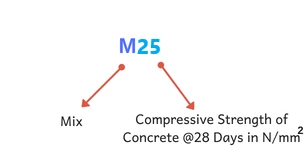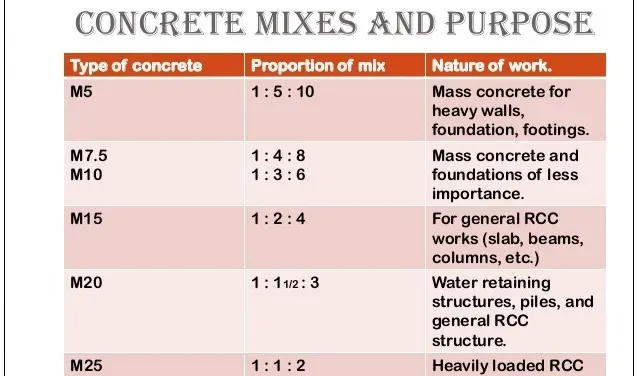Wednesday, October 4, 2023

# M25 Concrete Ratio (1:1:2) : Uses & Materials Required

M25 concrete ratio is 1:1:2, which indicates the ratio of cement : sand : aggregate.

✔ It simply means that if 1 kg is employed for making Concrete, you should use 1 kg sand and 2 kg aggregate. ( i.e. 1 part cement: 1 part sand: 2 parts aggregate )

✔ In M25 concrete,“M” stands for Mix ratio of cement:sand: aggregate  &  “25 “Stands for Compressive Strength @ 28 Days in N/mm².

✔ M25 Concrete is nominal Concrete that can be skillfully prepared using  Indian Standard IS 456:2000.

✔ Normally, about 38% of water is required for the complete hydration of Concrete.

As per Indian Standard IS 456:2000, for nominal mix ( i.e. M10, M15…, M25);  the w/c ratio varies from 0.4 to 0.6.

For M25 Concrete, commonly w/c ratio of 0.4 to 0.45 is used.

### 1. Table1: M25 Concrete Ratio – Overview

 Code Indian Standard IS 456:2000 Ratio 1:1:2 Compressive Strength 25 MPa { Note: MPa = N/mm²} Uses Used in RCC works like; a. Used in the construction of rigid pavements with high traffic. b. Used to construct structural members like slabs, beams, columns, etc. of bridges and buildings. ( Commonly used for heavy structures or work) Grade Category Standard Grade ( Nominal Mix ) Required  Water Cement Ratio a. For high-quality concrete works; 0.4 w/c ratio is specified. b. Minimum water-cement ratio: 0.40 c. Maximum water-cement ratio: 0.45 d. If plasticizers or superplasticizers are used, the w/c ratio may vary between 0.35 and 0.4.

### 2. M25 Concrete Ratio Uses

This is commonly used for medium to large-scale constructions. Some common uses of the M25 Concrete Ratio are:✔ Used in the construction of rigid pavements with high traffic.

✔ Used to construct structural members like slabs, beams, columns, bridges, etc.

✔ Used in constructing retaining walls where heavy loads have to be retained.

### 3. Cement Sand Aggregate Calculation For 1 m³ M25 Concrete Ratio

Data per 1 m³

 Material Volume Required (m3) Bag / Farma Materials Required in kg Cement 0.385 11.1 Bags 554.4 Kg Fine Aggregate 0.385 11 Farma 619.85 Kg Coarse Aggregate 0.77 22 Farma 1359.82 Kg Water 0.23 230kg ( Average )

 Things To Know!! Density of = 1440 kg/m3 Density of = 1450-1500 Kg/m3 Density of Aggregate = 1450-1550 Kg/m3 Weight of Cement in 1 Bag = 50 Kg The volume of 1 Bag of Cement in Cubic meter = 0.03472 m3

Now, Taking

Grade of Concrete = M25 Concrete

Mix Ratio = 1:1:2

Total sum of ratio =1+1+2 =4

Wet volume of Concrete = 1 m3

To get dry volume, wet volume is increased by 54%

Dry volume of concrete = 1 + 0.54 = 1.54 m³

#### i. Cement Quantity Calculation

Quantity of cement = (Own Ratio/ Total sum of ratio) * Dry Volume

= ( 1/4 ) * 1.54

=  0.385 m³

Cement in kg = volume of cement * density of cement

= 0.385 * 1440

= 554.4 kg

#### ii. Sand Quantity Calculation

Quantity of = (Own Ratio/ Total sum of ratio) * Dry Volume

= ( 1/4 ) * 1.54

=  0.385 m³

#### iii. Aggregate Quantity Calculation

Quantity of = (Own Ratio/ Total sum of ratio) * Dry Volume

= ( 2/4 ) * 1.54

=  0.77 m³

#### iv. Water Quantity Calculation

Minimum amount of water required per m³ = 0.4*554.4 = 221.76 kg = 221.76 L

Maximum amount of water required per m³ = 0.45*554.4 = 249.48 kg = 249.48 L

### 4. References

1.

 Verified Article By Er. Madhu Krishna PoudelHe is a founder and lead author of Dream Civil International and his civil engineering research articles has been taken as source by world's top news and educational sites like USA Today, Time, The richest, Wikipedia, etc.
Latest Articles

Related Articles